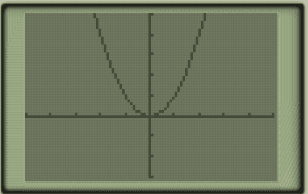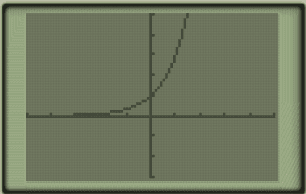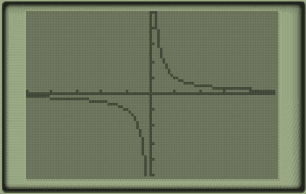# One to one functions

In algebra 1 and algebra 2, the topic of one to one functions often comes up. We know that if a function is one to one, it has an inverse, which can be useful knowledge.

# Graphical approaches:

Use horizontal and vertical line tests. Draw a vertical line through the graph and it should only intersect with function at one point. Draw a horizontal line through the graph and it should only intersect at one point.

example of a function that is not one to one (fails horizontal line test):example of a one to one function (passes both horizontal and vertical line tests):## Algebraic approach:

Algebraically, how to test for one to one:

Take g(x)= 1/x for example:

1.it’s one to one if g(a)=g(b)

1. plug in: g(a)=1/a and g(b)=1/b.
2. set them equal to each other: 1/a=1/b
3. if you multiply both sides by a, and both sides by b, you get a=b.
4. Thus, it’s one to one.
5. And we can confirm it graphically:Function above : 1/X